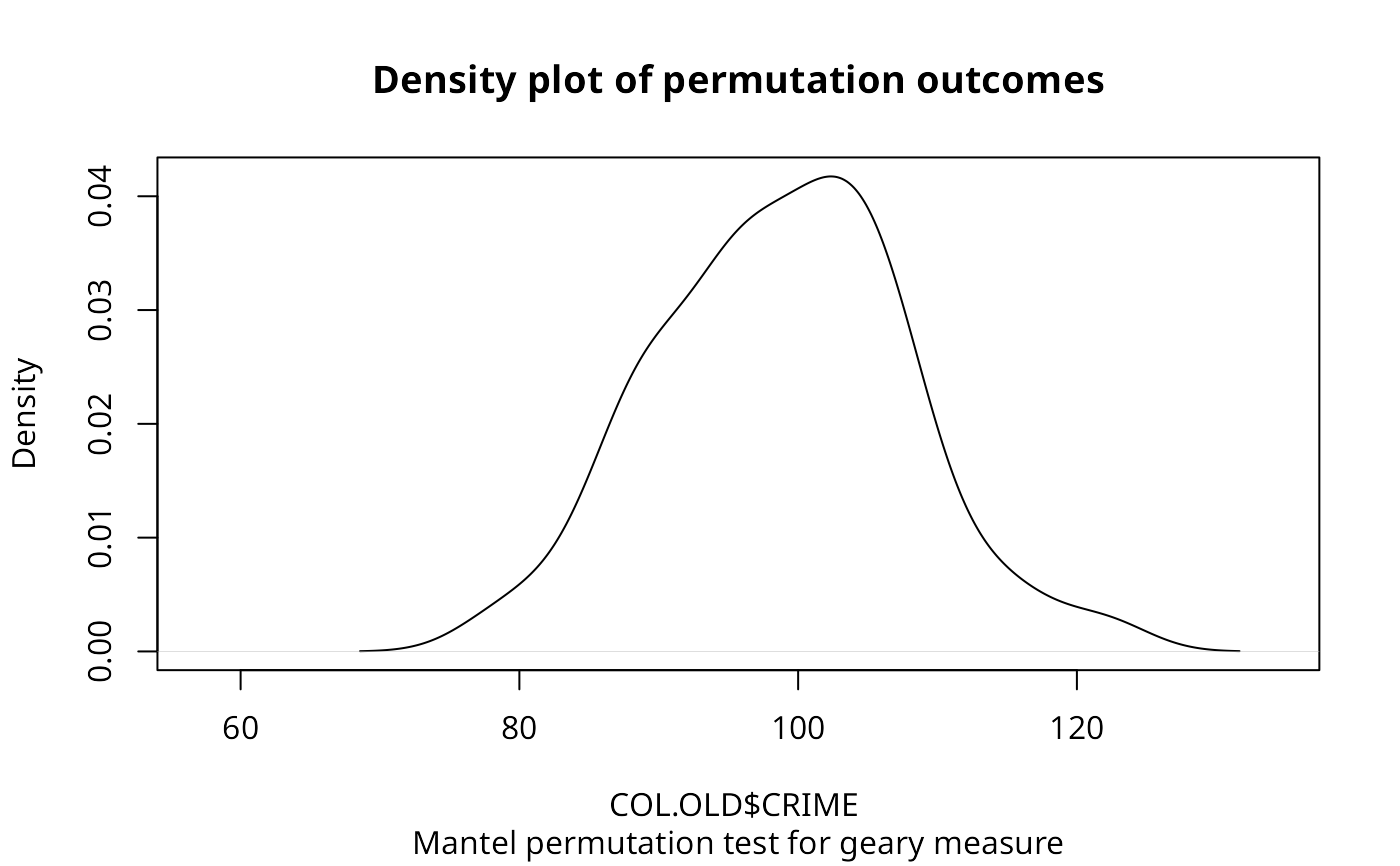A permutation test for the spatial general cross product statistic with Moran ($$C_{ij} = z_i z_j$$), Geary ($$C_{ij} = (z_i - z_j)^2$$), and Sokal ($$C_{ij} = |z_i - z_j|$$) criteria, for $$z_i = (x_i - \bar{x}) / \sigma_{x}$$. plot.mc.sim is a helper function to plot the outcomes of the permutation test.

sp.mantel.mc(var, listw, nsim, type = "moran", zero.policy = NULL,
alternative = "greater", spChk=NULL, return_boot=FALSE)
# S3 method for mc.sim
plot(x, xlim, xlab, main, sub, ..., ptype="density")

## Arguments

var

a numeric vector the same length as the neighbours list in listw

listw

a listw object created for example by nb2listw

nsim

number of permutations

type

"moran", "geary" or "sokal" criteria for similarity

zero.policy

default NULL, use global option value; if TRUE assign zero to the lagged value of zones without neighbours, if FALSE assign NA

alternative

a character string specifying the alternative hypothesis, must be one of "greater" (default), or "less".

spChk

should the data vector names be checked against the spatial objects for identity integrity, TRUE, or FALSE, default NULL to use get.spChkOption()

return_boot

return an object of class boot from the equivalent permutation bootstrap rather than an object of class htest

x

the object to be plotted

xlim

the range of the x axis

xlab

a title for the x axis

main

an overall title for the plot

sub

a sub title for the plot

ptype

either "density" or "hist"

...

further arguments passed through

## Value

A list with class htest and mc.sim containing the following components:

statistic

the value of the observed Geary's C.

parameter

the rank of the observed Geary's C.

alternative

a character string describing the alternative hypothesis.

method

a character string giving the method used.

data.name

a character string giving the name(s) of the data, and the number of simulations.

p.value

the pseudo p-value of the test.

res

nsim simulated values of statistic, final value is observed statistic

estimate

the mean and variance of the simulated distribution.

## Author

Roger Bivand Roger.Bivand@nhh.no

moran.mc, joincount.mc, geary.mc

## Examples

data(oldcol)
sim1 <- sp.mantel.mc(COL.OLD$CRIME, nb2listw(COL.nb), nsim=99, type="geary", alternative="less") sim1 #> #> Mantel permutation test for geary measure #> #> data: COL.OLD$CRIME
#> weights: nb2listw(COL.nb)
#> number of simulations + 1: 100
#>
#> statistic = 51.927, observed rank = 1, p-value = 0.01
#> alternative hypothesis: less
#> sample estimates:
#> mean of permutations   sd of permutations
#>            98.852997             8.934135
#>
plot(sim1)sp.mantel.mc(COL.OLD$CRIME, nb2listw(COL.nb), nsim=99, type="sokal", alternative="less") #> #> Mantel permutation test for sokal measure #> #> data: COL.OLD$CRIME
#> weights: nb2listw(COL.nb)
#> number of simulations + 1: 100
#>
#> statistic = 36.695, observed rank = 1, p-value = 0.01
#> alternative hypothesis: less
#> sample estimates:
#> mean of permutations   sd of permutations
#>            56.418584             3.227064
#>
sp.mantel.mc(COL.OLD$CRIME, nb2listw(COL.nb), nsim=99, type="moran") #> #> Mantel permutation test for moran measure #> #> data: COL.OLD$CRIME
#> weights: nb2listw(COL.nb)
#> number of simulations + 1: 100
#>
#> statistic = 24.526, observed rank = 100, p-value = 0.01
#> alternative hypothesis: greater
#> sample estimates:
#> mean of permutations   sd of permutations
#>           -0.3401381            4.5579226
#>